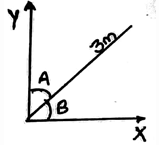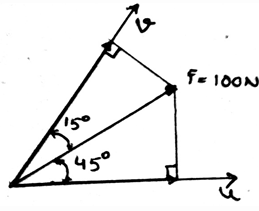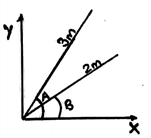Courses

# Test: Frictional Forces On Flat Belts

## 15 Questions MCQ Test Engineering Mechanics | Test: Frictional Forces On Flat Belts

Description
This mock test of Test: Frictional Forces On Flat Belts for Mechanical Engineering helps you for every Mechanical Engineering entrance exam. This contains 15 Multiple Choice Questions for Mechanical Engineering Test: Frictional Forces On Flat Belts (mcq) to study with solutions a complete question bank. The solved questions answers in this Test: Frictional Forces On Flat Belts quiz give you a good mix of easy questions and tough questions. Mechanical Engineering students definitely take this Test: Frictional Forces On Flat Belts exercise for a better result in the exam. You can find other Test: Frictional Forces On Flat Belts extra questions, long questions & short questions for Mechanical Engineering on EduRev as well by searching above.
QUESTION: 1

### ____________ is the phenomena that resist the movement of the two surfaces in contact, in some of the cases it could be the belts and the rolling cylinders.

Solution:

The friction is the phenomena that defines that there is a resistance which is present there between the two surfaces. The two surfaces are in contact and the friction applies at that surface only, resisting the motion of the surface.

QUESTION: 2

### The frictional force in the belts always acts ____________ to the surface of the application of the friction.

Solution:

The friction is the phenomena that defines that there is a resistance which is present there between the two surfaces. This friction is applied tangentially to the surfaces in contact. Thus the main thing is that the forces on both of the surfaces act tangential to each other.

QUESTION: 3

### Vector shown in the figure below have a length of 3m and the angles shown A and B are 60 and 30 degrees each. Calculate the X-axis and Y-axis components.Solution:

The sine and the cosine components of the given vectors considering the angle B as the only angle of consideration comes 1.5m and 2.59m.

QUESTION: 4

What is B in the equation T2 = T1eµB ?

Solution:

For solving of the unknown tension in the belts, T2 = T1eµB equation is used. In this the R.H.S tension is the maximum tension of the two tensions. While the other one is the smaller one. And the µ is coefficient of friction between the belt and the surface. And the B is the angle of belt to the surface in radians.

QUESTION: 5

Dry friction in the belt is also called ___________

Solution:

The dry friction is acted upon the surfaces. Whatever may be the surfaces. And they are tangential to each other. As we know the friction is the phenomena that defines that there is a resistance which is present there between the two surfaces. The dry friction is also termed as the Coulomb friction as it was given by C.A. Coulomb.

QUESTION: 6

For making the equilibrium equations for the belt the normal forces that are being acted over them are in which direction in the free body diagrams?

Solution:

As the loads are being acting in the downward direction. Thus to make the forces balance, the normal forces act in the vertically upward direction. As we know that when there is no lubricating fluid present between the surfaces in contact, the dry friction occurs. This friction magnitude is taken out from these normal forces

QUESTION: 7

Which one is not the condition for the equilibrium in free body diagram for the belts as considered for calculation of the normal forces, consider all forces to be straight and linear?

Solution:

For the equilibrium in the three dimensional system of axis we have all the conditions true as, ∑Fx=0, ∑Fy=0 and ∑Fz=0. Also we have the summation of the forces equal to zero. Which is not a non-zero value.

QUESTION: 8

We first make equilibrium equations of the belts by considering all the three dimensional forces acting on the section chosen and then the free body diagram is made and solved.

Solution:

We first make the free body diagram and then we make the equilibrium equations to satisfy the given conditions. This helps us to solve the question easily. As this reduces the part of imagination and increases accuracy too.

QUESTION: 9

We show the net forces acting on the belts by the help of __________ forces.

Solution:

The net forces acting on the body is shown by the help of the resultant forces. This is because the resultant forces have the sum of all the forces which are acting on the direction which is same. Thus the resultant forces are used to show the net forces acting in the body.

QUESTION: 10

There are main two types of forces which are being stated in the free body diagram of the belts, they are generally the resultant forces which are being acted over the body over which the belt is rolling. Which are they?

Solution:

The net forces acting on the body is shown by the help of the resultant forces. There are two types, first the frictional and the second is the normal. This is because the resultant forces have the sum of all the forces which are acting on the direction which is same.

QUESTION: 11

Determine the magnitude of the projection of the vector force F = 100N acting over a particular point on the belt, onto the v axis, from the figure given below.Solution:

The component of the force in the v axis, it is equal to 100cos(15˚). This is the application of the triangle over the figure. Try to resolve the components of the given force. It will be easy. This is one of the simplest example of solving for the forces acting over the belts.

QUESTION: 12

The solving for the unknown forces in the belts requires vector math. So if a vector is multiplied by a scalar in the belt system of forces then_________

Solution:

If a vector is multiplied by a scalar then its magnitude is increased by amount of that scalar’s magnitude. When multiplied by a negative scalar it going to change the directional sense of the vector. Vector math is unchanged throughout.

QUESTION: 13

All the vector quantities in the solving of the unknown in the belt force system obey _____________

Solution:

All the vectors quantities obey parallelogram law of addition. Two vectors A and B (can be called as component vectors) are added to form a resultant vector. R = A+B.

QUESTION: 14

For solving of the unknown tension in the belts, which of the following equation is used?

Solution:

For solving of the unknown tension in the belts, T2 = T1eµB equation is used. In this the R.H.S tension is the maximum tension of the two tensions. While the other one is the smaller one. And the µ is coefficient of friction between the belt and the surface.

QUESTION: 15

Shown as in the figure below, A=60 degree and B=30 degree. Calculate the total length obtained by adding the x-axis component of both the vectors.Solution:

After getting the cosine components of the given vectors we obtain the total length of the x-axis components to be 3cos60 + 2cos30 = 3.23.